# RD Sharma Solutions for Class 6 Maths Chapter 2: Playing with Numbers Objective Type Questions

Chapter 2 explains the properties of numbers, prime and composite numbers to the students. The objective-type questions which are available in the RD Sharma textbook help students self analyse their knowledge about the concepts covered. The experts in Mathematics provide RD Sharma Solutions Class 6 with the primary aim of assisting students to ace the exam. RD Sharma Solutions for Class 6 Maths Chapter 2 Playing with Numbers Objective Type Questions PDF is available here.

## RD Sharma Solutions for Class 6 Maths Chapter 2: Playing with Numbers Objective Type Questions Download PDF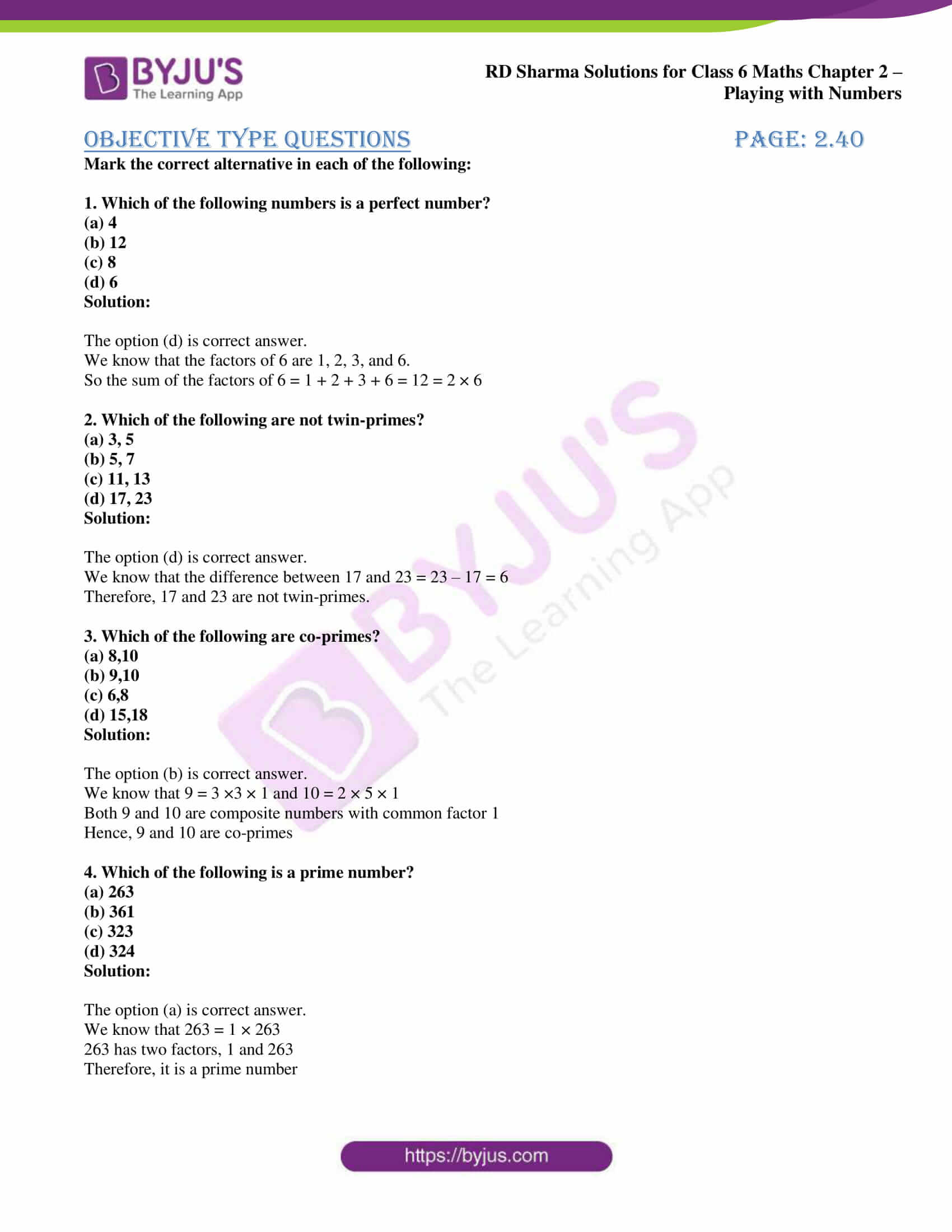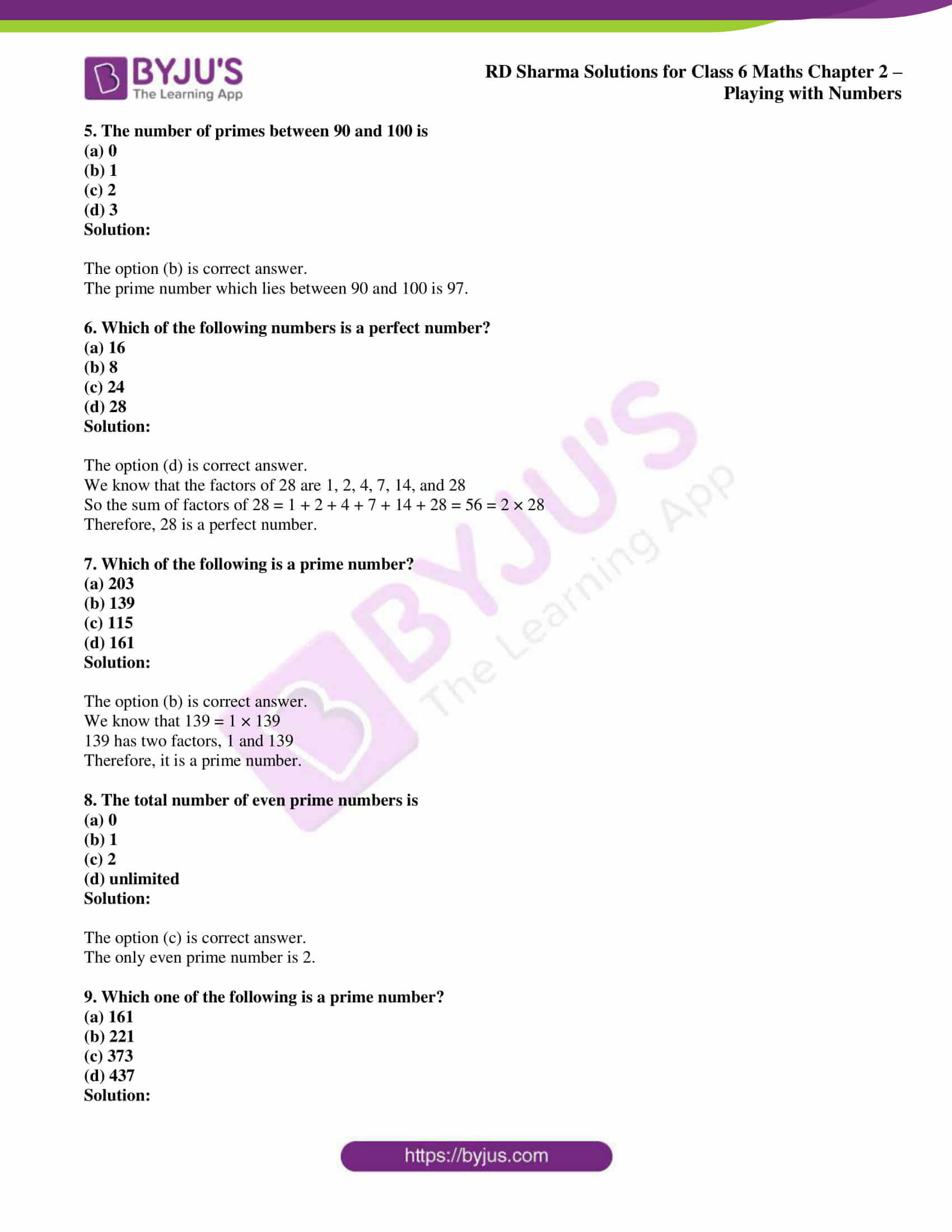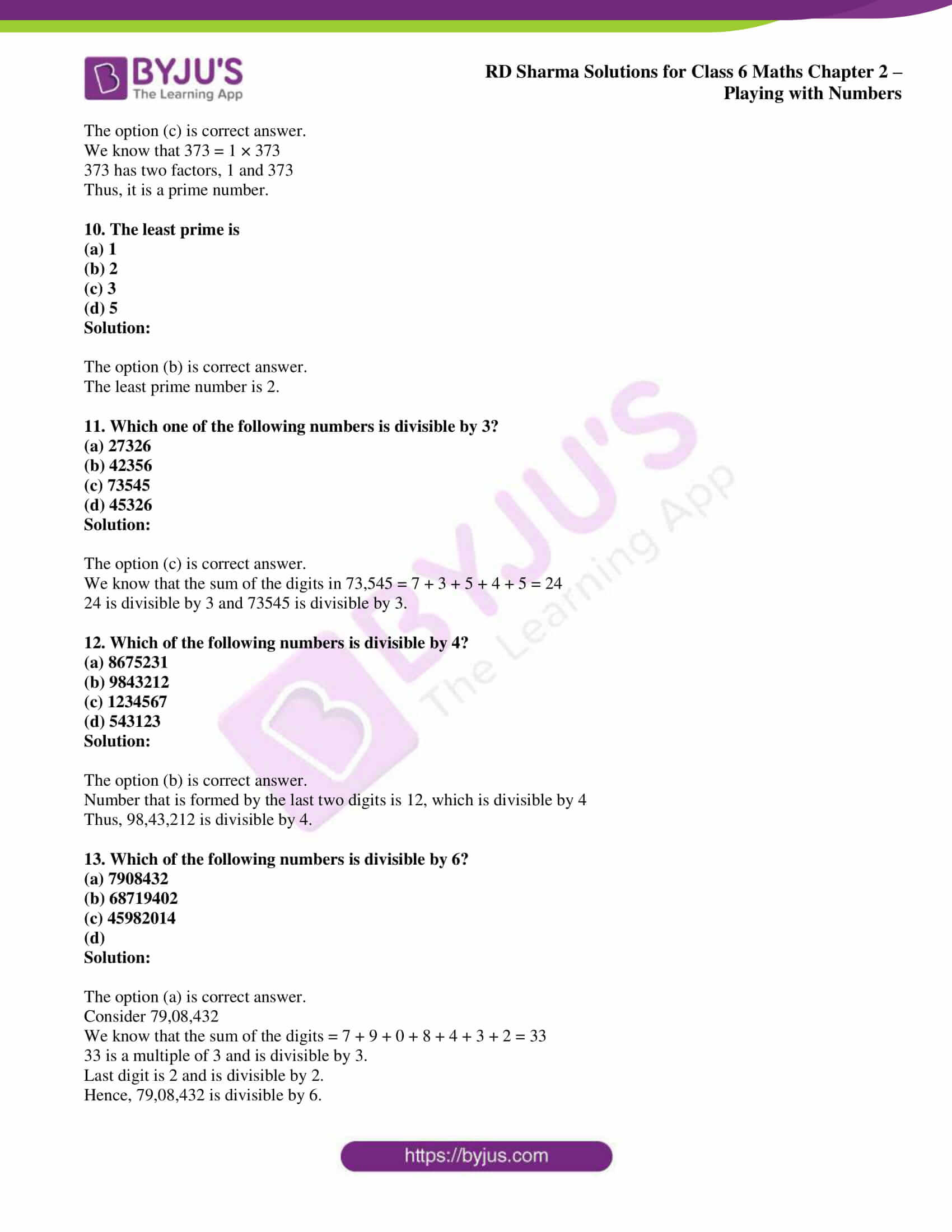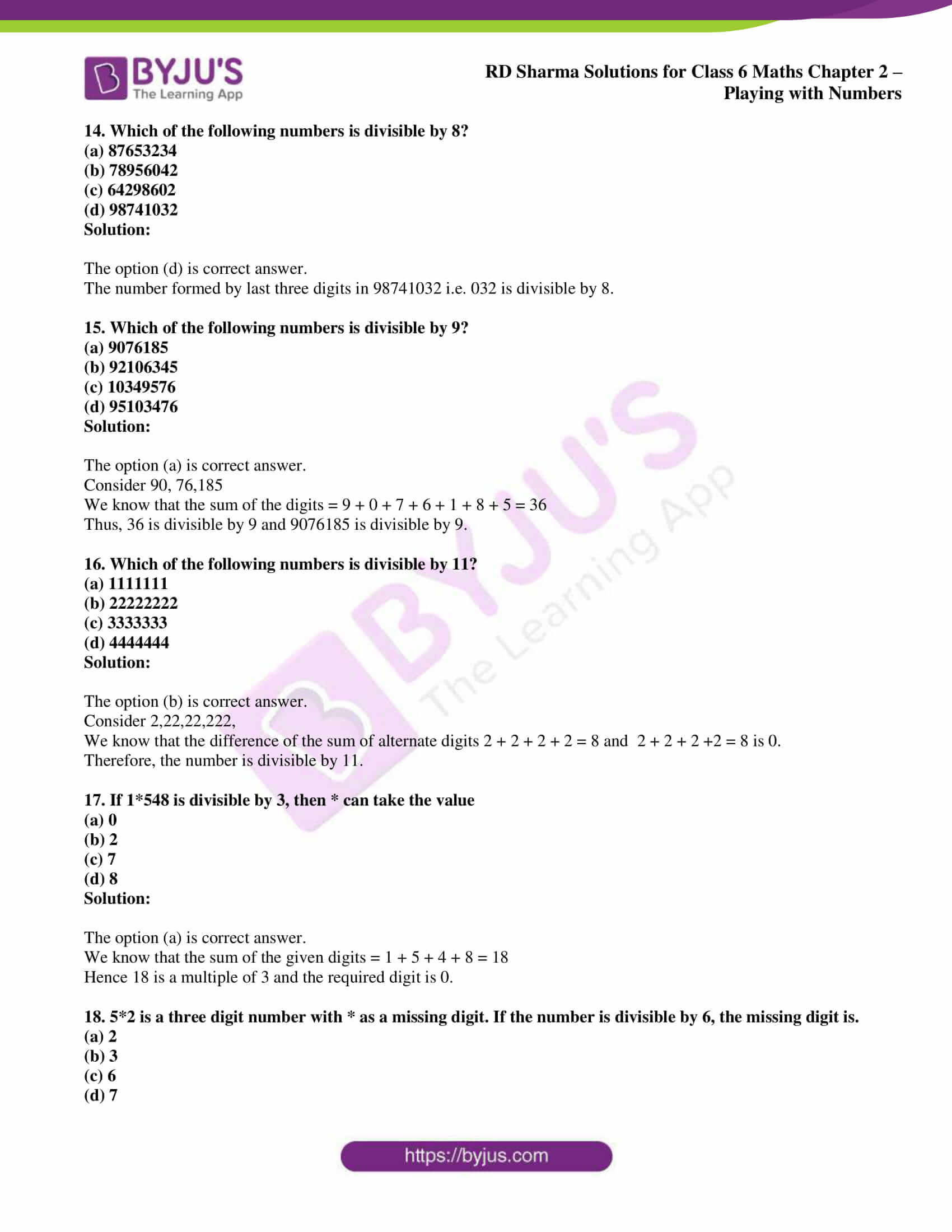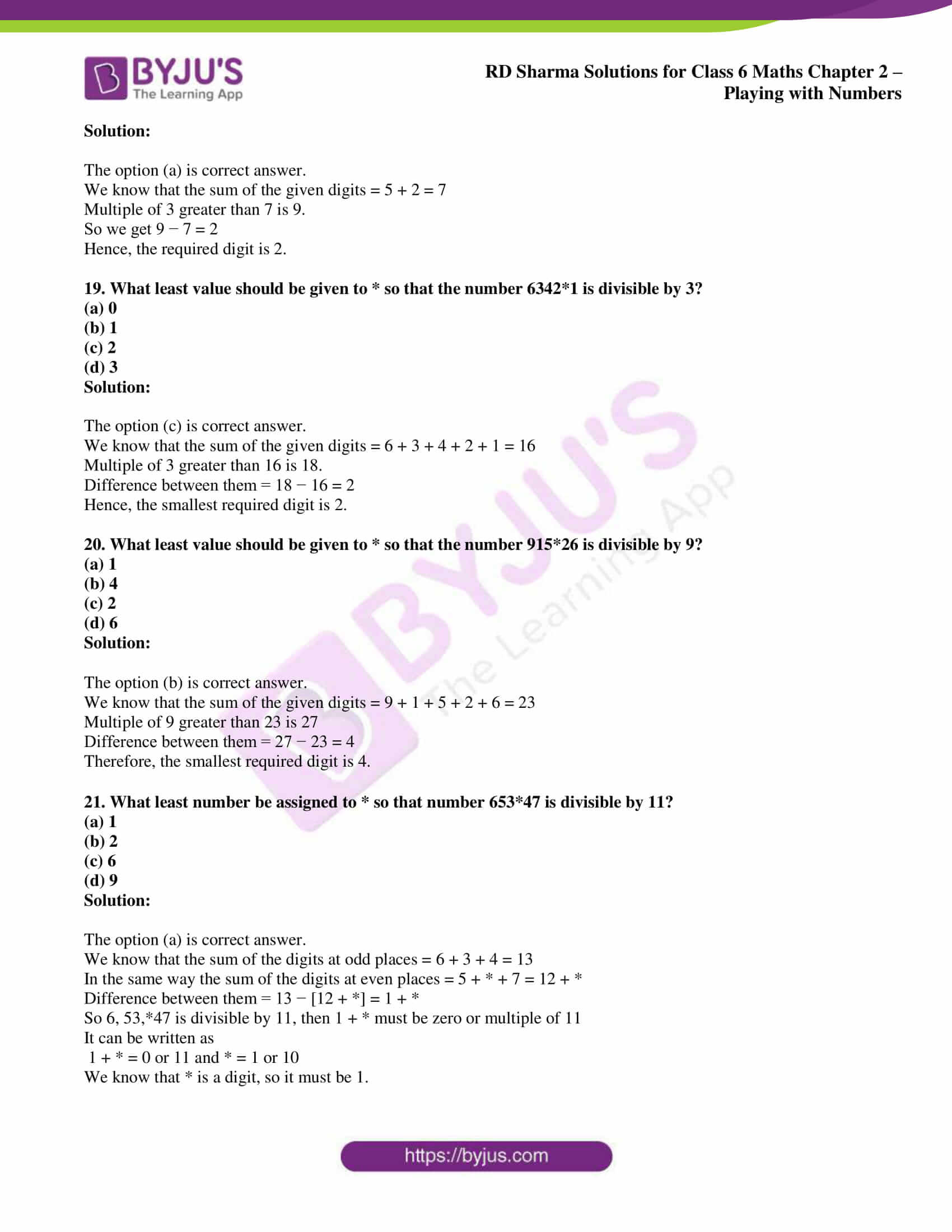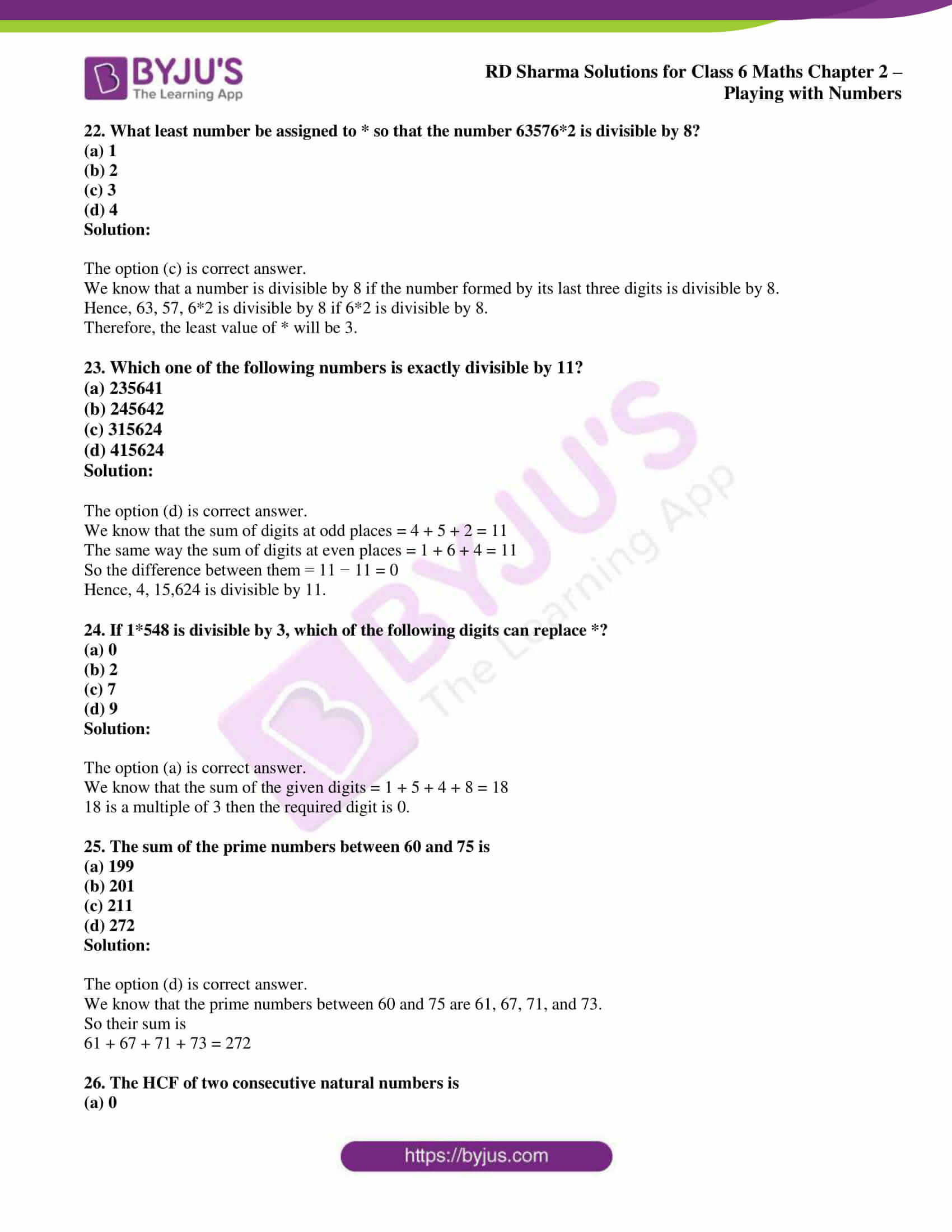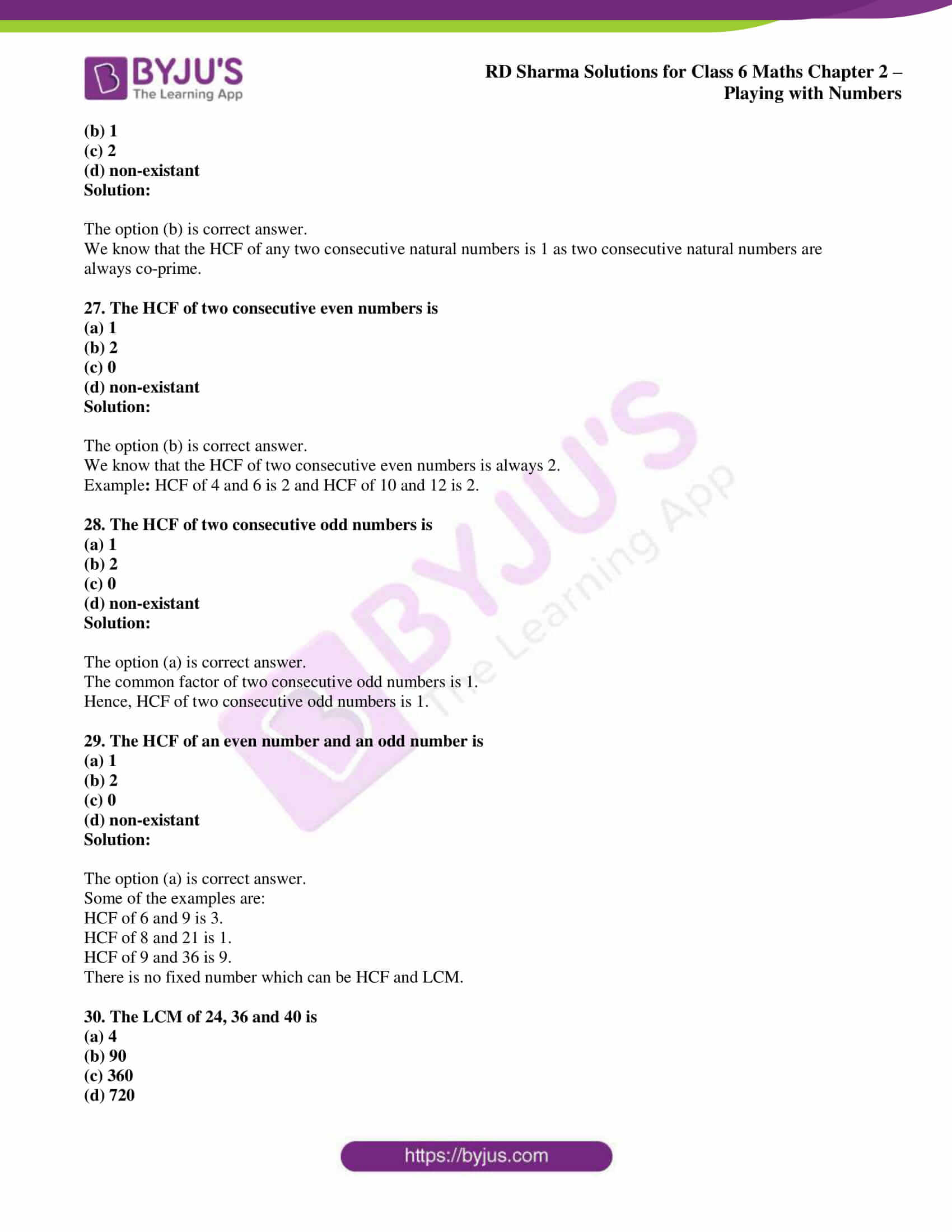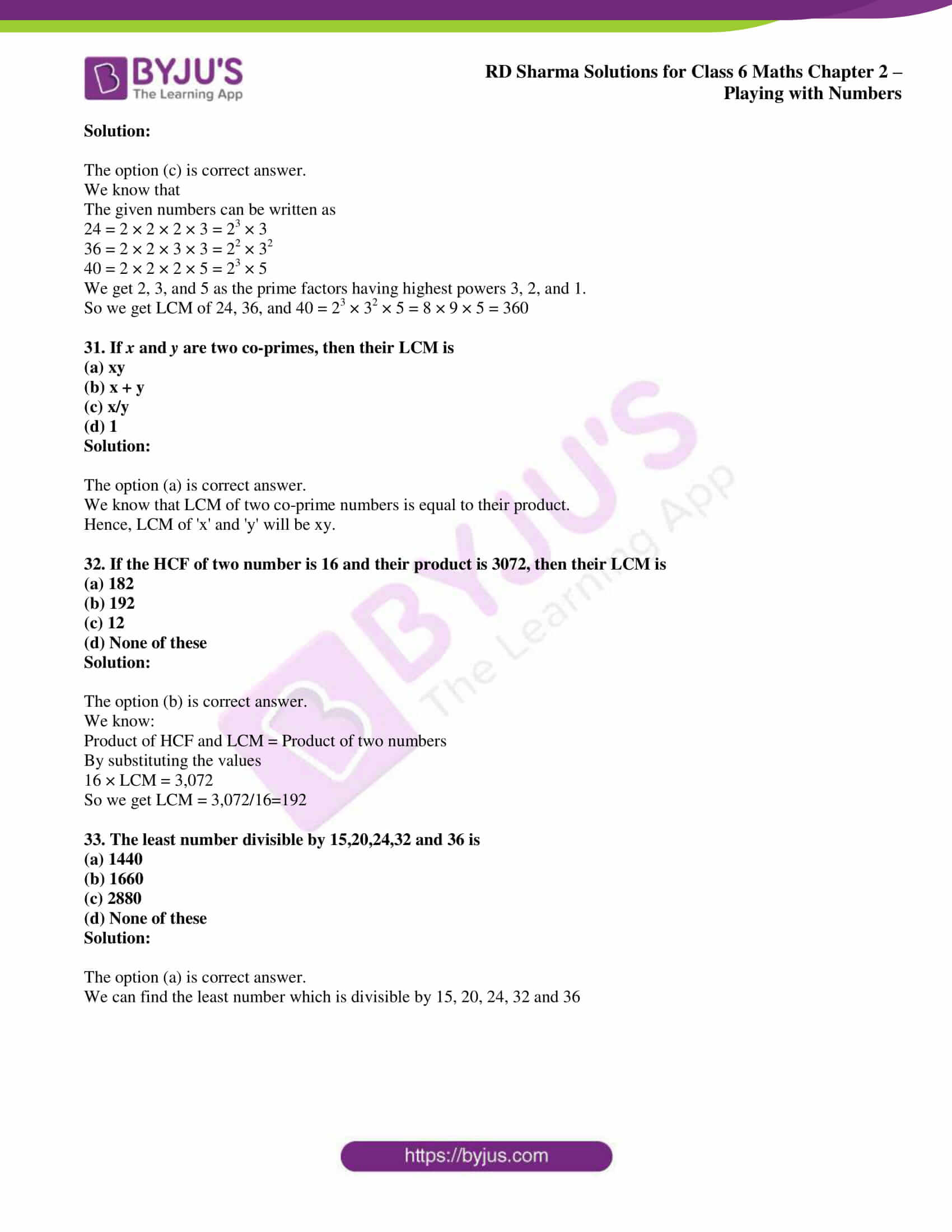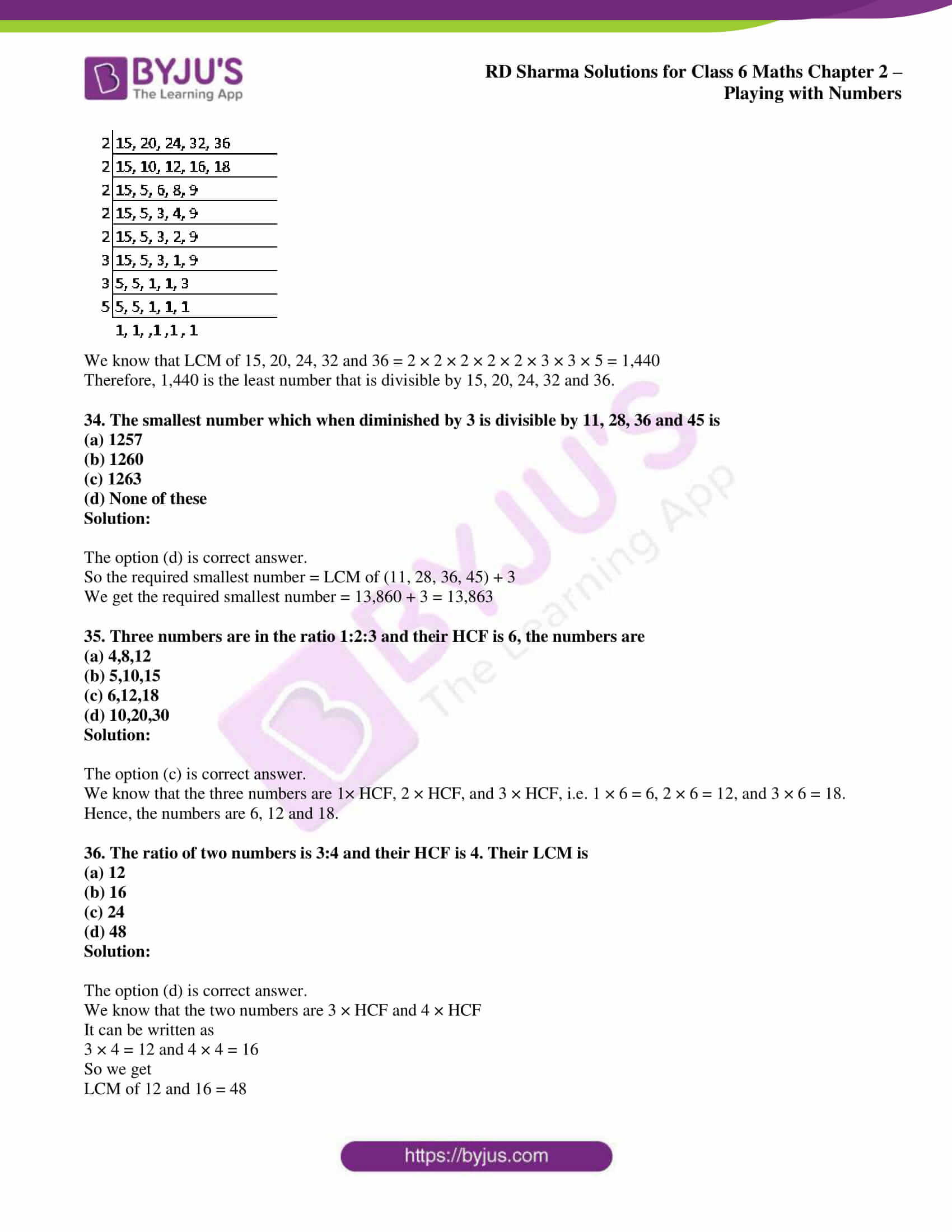### Access answers to Maths RD Sharma Solutions for Class 6 Chapter 2: Playing with Numbers Objective Type Questions

Mark the correct alternative in each of the following:

1. Which of the following numbers is a perfect number?
(a) 4
(b) 12
(c) 8
(d) 6

Solution:

The option (d) is correct answer.

We know that the factors of 6 are 1, 2, 3, and 6.

So the sum of the factors of 6 = 1 + 2 + 3 + 6 = 12 = 2 × 6

2. Which of the following are not twin-primes?
(a) 3, 5
(b) 5, 7
(c) 11, 13
(d) 17, 23

Solution:

The option (d) is correct answer.

We know that the difference between 17 and 23 = 23 – 17 = 6

Therefore, 17 and 23 are not twin-primes.

3. Which of the following are co-primes?
(a) 8,10
(b) 9,10
(c) 6,8
(d) 15,18

Solution:

The option (b) is correct answer.

We know that 9 = 3 ×3 × 1 and 10 = 2 × 5 × 1

Both 9 and 10 are composite numbers with common factor 1

Hence, 9 and 10 are co-primes

4. Which of the following is a prime number?
(a) 263
(b) 361
(c) 323
(d) 324

Solution:

The option (a) is correct answer.

We know that 263 = 1 × 263

263 has two factors, 1 and 263

Therefore, it is a prime number

5. The number of primes between 90 and 100 is
(a) 0
(b) 1
(c) 2
(d) 3

Solution:

The option (b) is correct answer.

The prime number which lies between 90 and 100 is 97.

6. Which of the following numbers is a perfect number?
(a) 16
(b) 8
(c) 24
(d) 28

Solution:

The option (d) is correct answer.

We know that the factors of 28 are 1, 2, 4, 7, 14, and 28

So the sum of factors of 28 = 1 + 2 + 4 + 7 + 14 + 28 = 56 = 2 × 28

Therefore, 28 is a perfect number.

7. Which of the following is a prime number?
(a) 203
(b) 139
(c) 115
(d) 161

Solution:

The option (b) is correct answer.

We know that 139 = 1 × 139

139 has two factors, 1 and 139

Therefore, it is a prime number.

8. The total number of even prime numbers is
(a) 0
(b) 1
(c) 2
(d) unlimited

Solution:

The option (c) is correct answer.

The only even prime number is 2.

9. Which one of the following is a prime number?
(a) 161
(b) 221
(c) 373
(d) 437

Solution:

The option (c) is correct answer.

We know that 373 = 1 × 373

373 has two factors, 1 and 373

Thus, it is a prime number.

10. The least prime is
(a) 1
(b) 2
(c) 3
(d) 5

Solution:

The option (b) is correct answer.

The least prime number is 2.

11. Which one of the following numbers is divisible by 3?
(a) 27326
(b) 42356
(c) 73545
(d) 45326

Solution:

The option (c) is correct answer.

We know that the sum of the digits in 73,545 = 7 + 3 + 5 + 4 + 5 = 24

24 is divisible by 3 and 73545 is divisible by 3.

12. Which of the following numbers is divisible by 4?
(a) 8675231
(b) 9843212
(c) 1234567
(d) 543123

Solution:

The option (b) is correct answer.

Number that is formed by the last two digits is 12, which is divisible by 4

Thus, 98,43,212 is divisible by 4.

13. Which of the following numbers is divisible by 6?
(a) 7908432
(b) 68719402
(c) 45982014
(d)

Solution:

The option (a) is correct answer.

Consider 79,08,432

We know that the sum of the digits = 7 + 9 + 0 + 8 + 4 + 3 + 2 = 33

33 is a multiple of 3 and is divisible by 3.

Last digit is 2 and is divisible by 2.

Hence, 79,08,432 is divisible by 6.

14. Which of the following numbers is divisible by 8?
(a) 87653234
(b) 78956042
(c) 64298602
(d) 98741032

Solution:

The option (d) is correct answer.

The number formed by last three digits in 98741032 i.e. 032 is divisible by 8.

15. Which of the following numbers is divisible by 9?
(a) 9076185
(b) 92106345
(c) 10349576
(d) 95103476

Solution:

The option (a) is correct answer.

Consider 90, 76,185

We know that the sum of the digits = 9 + 0 + 7 + 6 + 1 + 8 + 5 = 36

Thus, 36 is divisible by 9 and 9076185 is divisible by 9.

16. Which of the following numbers is divisible by 11?
(a) 1111111
(b) 22222222
(c) 3333333
(d) 4444444

Solution:

The option (b) is correct answer.

Consider 2,22,22,222,

We know that the difference of the sum of alternate digits 2 + 2 + 2 + 2 = 8 and  2 + 2 + 2 +2 = 8 is 0.

Therefore, the number is divisible by 11.

17. If 1*548 is divisible by 3, then * can take the value
(a) 0
(b) 2
(c) 7
(d) 8

Solution:

The option (a) is correct answer.

We know that the sum of the given digits = 1 + 5 + 4 + 8 = 18

Hence 18 is a multiple of 3 and the required digit is 0.

18. 5*2 is a three digit number with * as a missing digit. If the number is divisible by 6, the missing digit is.
(a) 2
(b) 3
(c) 6
(d) 7

Solution:

The option (a) is correct answer.

We know that the sum of the given digits = 5 + 2 = 7

Multiple of 3 greater than 7 is 9.

So we get 9 − 7 = 2

Hence, the required digit is 2.

19. What least value should be given to * so that the number 6342*1 is divisible by 3?
(a) 0
(b) 1
(c) 2
(d) 3

Solution:

The option (c) is correct answer.

We know that the sum of the given digits = 6 + 3 + 4 + 2 + 1 = 16

Multiple of 3 greater than 16 is 18.

Difference between them = 18 − 16 = 2

Hence, the smallest required digit is 2.

20. What least value should be given to * so that the number 915*26 is divisible by 9?
(a) 1
(b) 4
(c) 2
(d) 6

Solution:

The option (b) is correct answer.

We know that the sum of the given digits = 9 + 1 + 5 + 2 + 6 = 23

Multiple of 9 greater than 23 is 27

Difference between them = 27 − 23 = 4

Therefore, the smallest required digit is 4.

21. What least number be assigned to * so that number 653*47 is divisible by 11?
(a) 1
(b) 2
(c) 6
(d) 9

Solution:

The option (a) is correct answer.

We know that the sum of the digits at odd places = 6 + 3 + 4 = 13

In the same way the sum of the digits at even places = 5 + * + 7 = 12 + *

Difference between them = 13 − [12 + *] = 1 + *

So 6, 53,*47 is divisible by 11, then 1 + * must be zero or multiple of 11

It can be written as

1 + * = 0 or 11 and * = 1 or 10

We know that * is a digit, so it must be 1.

22. What least number be assigned to * so that the number 63576*2 is divisible by 8?
(a) 1
(b) 2
(c) 3
(d) 4

Solution:

The option (c) is correct answer.

We know that a number is divisible by 8 if the number formed by its last three digits is divisible by 8.

Hence, 63, 57, 6*2 is divisible by 8 if 6*2 is divisible by 8.

Therefore, the least value of * will be 3.

23. Which one of the following numbers is exactly divisible by 11?
(a) 235641
(b) 245642
(c) 315624
(d) 415624

Solution:

The option (d) is correct answer.

We know that the sum of digits at odd places = 4 + 5 + 2 = 11

The same way the sum of digits at even places = 1 + 6 + 4 = 11

So the difference between them = 11 − 11 = 0

Hence, 4, 15,624 is divisible by 11.

24. If 1*548 is divisible by 3, which of the following digits can replace *?
(a) 0
(b) 2
(c) 7
(d) 9

Solution:

The option (a) is correct answer.

We know that the sum of the given digits = 1 + 5 + 4 + 8 = 18

18 is a multiple of 3 then the required digit is 0.

25. The sum of the prime numbers between 60 and 75 is
(a) 199
(b) 201
(c) 211
(d) 272

Solution:

The option (d) is correct answer.

We know that the prime numbers between 60 and 75 are 61, 67, 71, and 73.

So their sum is

61 + 67 + 71 + 73 = 272

26. The HCF of two consecutive natural numbers is
(a) 0
(b) 1
(c) 2
(d) non-existant

Solution:

The option (b) is correct answer.

We know that the HCF of any two consecutive natural numbers is 1 as two consecutive natural numbers are always co-prime.

27. The HCF of two consecutive even numbers is
(a) 1
(b) 2
(c) 0
(d) non-existant

Solution:

The option (b) is correct answer.

We know that the HCF of two consecutive even numbers is always 2.

Example: HCF of 4 and 6 is 2 and HCF of 10 and 12 is 2.

28. The HCF of two consecutive odd numbers is
(a) 1
(b) 2
(c) 0
(d) non-existant

Solution:

The option (a) is correct answer.

The common factor of two consecutive odd numbers is 1.

Hence, HCF of two consecutive odd numbers is 1.

29. The HCF of an even number and an odd number is
(a) 1
(b) 2
(c) 0
(d) non-existant

Solution:

The option (a) is correct answer.

Some of the examples are:

HCF of 6 and 9 is 3.

HCF of 8 and 21 is 1.

HCF of 9 and 36 is 9.

There is no fixed number which can be HCF and LCM.

30. The LCM of 24, 36 and 40 is
(a) 4
(b) 90
(c) 360
(d) 720

Solution:

The option (c) is correct answer.

We know that

The given numbers can be written as

24 = 2 × 2 × 2 × 3 = 23 × 3

36 = 2 × 2 × 3 × 3 = 22 × 32

40 = 2 × 2 × 2 × 5 = 23 × 5

We get 2, 3, and 5 as the prime factors having highest powers 3, 2, and 1.

So we get LCM of 24, 36, and 40 = 23 × 32 × 5 = 8 × 9 × 5 = 360

31. If x and y are two co-primes, then their LCM is
(a) xy
(b) x + y
(c) x/y
(d) 1

Solution:

The option (a) is correct answer.

We know that LCM of two co-prime numbers is equal to their product.

Hence, LCM of ‘x’ and ‘y’ will be xy.

32. If the HCF of two number is 16 and their product is 3072, then their LCM is
(a) 182
(b) 192
(c) 12
(d) None of these

Solution:

The option (b) is correct answer.

We know:

Product of HCF and LCM = Product of two numbers

By substituting the values

16 × LCM = 3,072

So we get LCM = 3,072/16=192

33. The least number divisible by 15,20,24,32 and 36 is
(a) 1440
(b) 1660
(c) 2880
(d) None of these

Solution:

The option (a) is correct answer.

We can find the least number which is divisible by 15, 20, 24, 32 and 36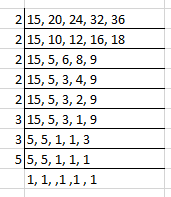We know that LCM of 15, 20, 24, 32 and 36 = 2 × 2 × 2 × 2 × 2 × 3 × 3 × 5 = 1,440

Therefore, 1,440 is the least number that is divisible by 15, 20, 24, 32 and 36.

34. The smallest number which when diminished by 3 is divisible by 11, 28, 36 and 45 is
(a) 1257
(b) 1260
(c) 1263
(d) None of these

Solution:

The option (d) is correct answer.

So the required smallest number = LCM of (11, 28, 36, 45) + 3

We get the required smallest number = 13,860 + 3 = 13,863

35. Three numbers are in the ratio 1:2:3 and their HCF is 6, the numbers are
(a) 4,8,12
(b) 5,10,15
(c) 6,12,18
(d) 10,20,30

Solution:

The option (c) is correct answer.

We know that the three numbers are 1× HCF, 2 × HCF, and 3 × HCF, i.e. 1 × 6 = 6, 2 × 6 = 12, and 3 × 6 = 18.

Hence, the numbers are 6, 12 and 18.

36. The ratio of two numbers is 3:4 and their HCF is 4. Their LCM is
(a) 12
(b) 16
(c) 24
(d) 48

Solution:

The option (d) is correct answer.

We know that the two numbers are 3 × HCF and 4 × HCF

It can be written as

3 × 4 = 12 and 4 × 4 = 16

So we get

LCM of 12 and 16 = 48